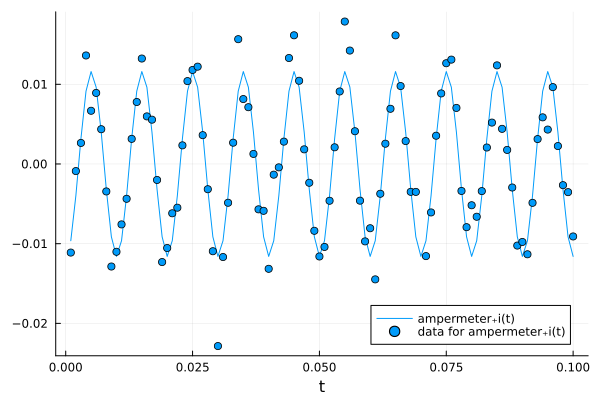# Custom Loss Functions

This tutorial assumes that you have read the getting started tutorial. By default JuliaSimModelOptimizer uses the l2loss, but this is not always the best choice. In this tutorial, will work with the same model as the getting started tutorial.

using JuliaSimModelOptimizer
using OrdinaryDiffEq
using ModelingToolkit
using ModelingToolkitStandardLibrary.Electrical
using ModelingToolkitStandardLibrary.Blocks: Sine
using DataSets
using Plots

function create_model(; C₁ = 3e-5, C₂ = 1e-6)
@variables t
@named resistor1 = Resistor(R = 5.0)
@named resistor2 = Resistor(R = 2.0)
@named capacitor1 = Capacitor(C = C₁)
@named capacitor2 = Capacitor(C = C₂)
@named source = Voltage()
@named input_signal = Sine(frequency = 100.)
@named ground = Ground()
@named ampermeter = CurrentSensor()

eqs = [connect(input_signal.output, source.V)
connect(source.p, capacitor1.n, capacitor2.n)
connect(source.n, resistor1.p, resistor2.p, ground.g)
connect(resistor1.n, capacitor1.p, ampermeter.n)
connect(resistor2.n, capacitor2.p, ampermeter.p)]

@named circuit_model = ODESystem(eqs, t,
systems = [
resistor1, resistor2, capacitor1, capacitor2,
source, input_signal, ground, ampermeter,
])
end
model = create_model()
sys = structural_simplify(model)
\begin{align} \frac{\mathrm{d} capacitor2_{+}v\left( t \right)}{\mathrm{d}t} =& capacitor1_{+}vˍt\left( t \right) \\ 0 =& - capacitor1_{+}i\left( t \right) - capacitor2_{+}i\left( t \right) + resistor1_{+}i\left( t \right) + resistor2_{+}i\left( t \right) \end{align}

However, in this tutorial, the data is noisier. Furthermore, the strength of the noise seems to depend on the absolute value of the current $i$ going through the ampermeter. The data can be read in using the dataset function. If you are using JuliaSimModelOptimizer locally you might have to follow these steps to make the dataset available.

data = dataset("juliasimtutorials/circuit_data_heterogeneous")
name = "juliasimtutorials/circuit_data_heterogeneous"
uuid = "0a1054b8-ab9c-49d2-893a-d71525018965"
description = "Data for the Model Optimizer loss tutorial."
type = "Blob"

[storage]
driver = "JuliaHubDataRepo"
bucket_region = "us-east-1"
bucket = "internaljuilahubdatasets"
credentials_url = "https://internal.juliahub.com/datasets/0a1054b8-ab9c-49d2-893a-d71525018965/credentials"
prefix = "datasets"
vendor = "aws"
type = "Blob"

[storage.juliahub_credentials]
auth_toml_path = "/home/github_actions/actions-runner-1/home/.julia/servers/internal.juliahub.com/auth.toml"

[[storage.versions]]
blobstore_path = "u1"
date = "2023-08-17T01:07:47.62+00:00"
size = 2776
version = 1


For heteroscedastic noise a weighted variant of l2loss outperforms the default l2loss. Specifically, we assume that the standard deviation of the measurement noise increases linearly as i increases. We can implement such a weighted loss ourselves using the err keyword of experiment. err should always be a function with two inputs, the solution of the model, at a certain point in the search space, and the data. Here the solution will always only contain the states and timepoints present in the dataset, e.g. state voltages will not be present in the solution. The dataset is transformed from a DataFrame to a Matrix. Both inputs are ordered in the same way, i.e. solution[i,j] corresponds to the same state and timepoint as dataset[i,j]. We can then easily implement losses using broadcasting.

function weighted_loss(solution, dataset)
sum(((solution .- dataset)./dataset).^2)
end
experiment = Experiment(data, sys; alg = Rodas4(), abstol=1e-6, reltol=1e-5)
@unpack capacitor2 = model
search_space = [capacitor2.C => (1.e-7, 1e-3)]
invprob = InverseProblem(experiment, sys, search_space)
alg = SingleShooting(maxiters = 10^4)
r = calibrate(invprob, alg)
Calibration result computed in 11 seconds and 983 milliseconds. Final objective value: 0.000930384.
Optimization ended with Success Return Code and returned.

┌──────────────┐
│ capacitor2₊C │
├──────────────┤
│   1.03056e-5 │
└──────────────┘


We see that this calibrated current fits the data well.

plot(experiment, invprob, r, show_data=true, legend=true)